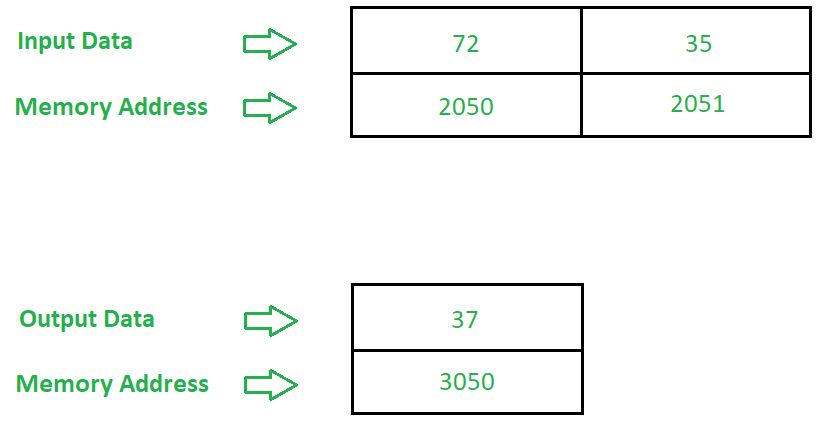# 8085 program to subtract two BCD numbers

• Last Updated : 18 May, 2018

Problem – Write an assembly language program in 8085 microprocessor to subtract two 8 bit BCD numbers.

Example –Algorithm –

2. Move the data from A to C
3. Move the data 99 in A
4. Subtract the contents of registers A and C
5. Increment the content of A by 1
6. Move the data from A to B
8. Add the contents of A and C and adjust it in BCD format by using DAA instruction
9. Store the result at memory address 3050
10. Stop

Program –

2000LDA 2051A <- 2051
2003MOV C, AC <- A
2004MVI A 99A <- 99
2006SUB CA = A – C
2007INR AA = A + 1
2008MOV B, AB <- A
2009LDA 2050A <- 2050
200CADD BA = A + B
200DDAAConvert the hexadecimal value to BCD value
200ESTA 30503050 <- A
2011HLTStop

Explanation –

1. LDA 2051 is used to load the data from address 2051 in A.
2. MOV C, A is used to move the data from A to C.
3. MVI A 99 is used to move the data to register A.
4. SUB C is used to subtract the contents of registers A and C.
5. INR A is used to increment the content of A by 1.
6. MOV B, A is used to move the data from A to B.
7. LDA 2050 is used to load the data from address 2050 in A.
8. ADD B is used to add the contents of registers A and B.
9. DAA is used to convert the hexadecimal value in Accumulator to BCD value.
10. STA 3050 is used to store the contents of A to 3050.
11. HLT is used end the program.
My Personal Notes arrow_drop_up